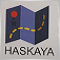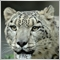8

I have a problem when programming in MQL4. It is a very strange problem. I am curious whether someone can tell me what is going on here.
Below I present two pieces of simplified code, code version 1 and code version 2. Both versions are identical with the only difference the fact that in code version 1 one line is deactivated with a comment sign (//).

// code version 1
if(condition)
{
A=....   ;   // calcuation of the value of A
B=....   ;   // calcuation of the value of B
C=....   ;   // calcuation of the value of C
//   TicketNumber=OrderSend(..using A, B and C..);
}

// code version 2
if(condition)
{
A=....   ;   // calcuation of the value of A
B=....   ;   // calcuation of the value of B
C=....   ;   // calcuation of the value of C
TicketNumber=OrderSend(..using A, B and C..);
}

When running the program with code version 1 then, when the condition is fulfilled, I see the results of A, B and C presented. The TicketNumber didn't get a new value. OK. When I do the same with code version 2, under the same circumstances, then the values of A, B and C are not presented, as if there are no alerts at all, but the order is sent.
My question: what is going on here, that with an activated OrderSend function the Alerts don't work anymore? The alerts are important to me because I use them to check the intermediate values of variables for checking the proper functioning of the program.1671

gcvdberg:

I have a problem when programming in MQL4. It is a very strange problem. I am curious whether someone can tell me what is going on here.
Below I present two pieces of simplified code, code version 1 and code version 2. Both versions are identical with the only difference the fact that in code version 1 one line is deactivated with a comment sign (//).

// code version 1
if(condition)
{
A=....   ;   // calcuation of the value of A
B=....   ;   // calcuation of the value of B
C=....   ;   // calcuation of the value of C
//   TicketNumber=OrderSend(..using A, B and C..);
}

// code version 2
if(condition)
{
A=....   ;   // calcuation of the value of A
B=....   ;   // calcuation of the value of B
C=....   ;   // calcuation of the value of C
TicketNumber=OrderSend(..using A, B and C..);
}

When running the program with code version 1 then, when the condition is fulfilled, I see the results of A, B and C presented. The TicketNumber didn't get a new value. OK. When I do the same with code version 2, under the same circumstances, then the values of A, B and C are not presented, as if there are no alerts at all, but the order is sent.
My question: what is going on here, that with an activated OrderSend function the Alerts don't work anymore? The alerts are important to me because I use them to check the intermediate values of variables for checking the proper functioning of the program.

```  bool ticket=OrderSend(Symbol(),OP_BUY,Ask,3,0,0,"BuySellComment",MagicNumber,0,CLR_NONE);882

Hello, try this

```switch(x)
{
case 'A':
Print("CASE A");
break;
case 'B':
case 'C':
Print("CASE B or C");
break;
default:
Print("NOT A, B or C");
break;
} ```